All courses delivered remotely. Instructor-led, live online.

# Power BI DAX Training Course

4.72
Learn Power BI DAX from our experienced trainers in our remote courses, live online.
HK\$3,200
10 max
1 day

## Power BI DAX Course Details

Price
HK\$3,200
Duration
1 day
Times
9am - 4pm ( approx )
Class Size (max)
10
Class Size (avg)
5
Reference Materials
Provided
CPD Hours
6 hours
Delivery
Instructor-led training
Live Online
16F & 17F
Kowloon
Hong Kong## Power BI Course Outlines

• Power BI Beginner
HK\$2,100
• Power BI Intermediate
HK\$3,200
HK\$3,200
• Power BI DAX
HK\$3,200
• DA-100 Power BI Certification
Other Courses
Other Courses
Course Rating4.72 5 Microsoft Power BI Training Courses
4.72 out of 5
35218 Student Reviews

## Skills Test

Try our short skills test and find out which course is right for you.## What do I need to know to attend?

• Use Power BI Desktop proficiently to source / model data and create basic tables, columns and measures with DAX
• Understanding the basic concepts of data modelling and how to perform related tasks in Power BI
• Have good general knowledge of simple DAX and understand the evaluation contexts (row, filter, context transition etc.)
• Advanced Excel skills is desired, knowledge of programming / scripting concepts will be an advantage
• Students should have attended our Power BI Advanced course.

## Power BI DAX Learning Outcomes

• Write expressions more confidently using DAX
• Work with a wider range of DAX functions
• Make use of tools to compliment creating and managing DAX

• Create more complex expressions using methods to build and prove an outcome
• Appreciate the concepts of data model and expression performance
• Use techniques to optimise an expression for better efficiency

## Power BI DAX Course Content

• Course Introduction
• What is DAX
• DAX Functions
• DAX Contexts
• What is Vertipaq
• DAX efficiency
• DAX references
• DAX best practices

• Recap of Contexts
• Introduction to Contexts
• Recap of DAX Contexts
• The Row Context
• The Filter Context
• Measures and Calculated Columns
• Dataset for exercises – Computer Store sales
• Filter and Row Context in a measure
• The CALCULATE function
• Context Transition
• Filter Expressions of CALCULATE
• Rules of Evaluation
• CALCULATE and FILTER

• Contexts in Calculated Columns
• Introduction to Columns
• Row Context in Calculated Columns
• Filter Context in Calculated Columns
• Relationships in Row Contexts
• The RELATED function
• The RELATEDTABLE function
• Using RELATEDTABLE in a column
• CALCULATED in row contexts
• Row to Filter Context
• Transformation Whiteboard
• Exercise on row to filter contexts
• Duplicates in the transformation
• The LOOKUPVALUE function

• Row Contexts in Measures
• Introduction to Row Contexts
• Row Contexts in Measures
• Simple iterator in a measure
• Row Context and a related table
• Some more calculations exercise
• Re-using measures
• Implicit CALCULATE in a measure
• An alternative approach using variables

• Functions and FILTER
• Introduction to DAX functions
• Time Intelligence functions
• Date (Dimension) tables
• Using in-built TOTALYTD
• Date modifier functions
• Using date modifier DATESBETWEEN
• Using date modifier DATESYTD
• The magic behind Date Table functions
• The case for simplicity – MAX or LASTDATE
• Understanding the FILTER function
• Contexts in the FILTER function
• The filter context in functions
• Naked column references
• Simple filter expression
• Comparing a value in the filter context
• The value of VALUES

• Table Functions
• Introduction to Table functions
• Summarising tables
• Using SUMMARIZE alone
• The problem with SUMMARIZE
• Using SUMMARIZECOLUMNS as a better alternative
• A summary table in a measure

• Practical DAX
• Introduction to DAX calculations
• Comparing functions – min/max and time intelligence
• Debugging with DAX
• Alternative patterns
• The Quick Measure version
• The KEEPFILTERS function
• The TREATAS function
• A few ORs or TREATAS
• Using TREATAS and KEEPFILTERS
• Using TREATAS for virtual relationships

• DAX Tools
• Use of third party tools
• Introducing DAX Studio
• What is DAX Studio
• The DAX Studio Interface
• How DAX Studio connects to the data
• Viewing data model information
• Creating a table query
• Ordering a query result
• A query returning a scalar
• Defining a Measure
• Declaring a variable
• Incoming filter context

• DAX Performance
• Introduction to DAX Performance
• The basics of the Vertipaq engine
• Where is Vertipaq
• A database of columns
• Compression of a column
• Identifying column compression
• Understanding the TABLE_ID column
• Power Query data statistics
• Data Model Normalisation
• Introduction to the Query Plan
• Overview of the engines
• The Formula Engine
• The Storage Engine
• Capturing a query
• Special process to capture Power BI queries
• Understanding the query information from the capture

• DAX Optimisation
• Introduction to optimising DAX
• Clear the Cache
• Server Timings
• Explaining the Server Timings
• Steps to improve performance
• Redefine a measure
• The datacache
• Optimisation by the Vertipaq engine
• The CallBackDataID function
• Reducing CallBackDataID instances
• Optimising a Query in the Storage Engine
• Viewing the Query Plan
• Comparing two statistical functions

• Extended Topics:
• Table Joins
• Introduction to Table Joins
• The Inner Join
• A join on unrelated tables – TREATAS
• The Outer Join
• GENERATE for Joins

• Nested Row Context
• Introduction to Nested Rows
• How Nested Rows work
• The EARLIER function
• The EARLIEST function
• Create a nested row in a column
• How to avoid complex nesting expressions
• Using variables to store values before nesting
• Avoid iterators where possible
• Further improve the efficiency of an expression

• Ranking
• Introduction to Ranking
• Arguments in RANKX
• Create a calculated column for ranking
• Sort order of ranking
• Definitions when ranking ties
• Breaking Ties Manually
• Creating a measure for ranking
• Adjustments to make the RANKX measure work

### What is Remote Training?

••Instructor-led Training
••Innovative Content
•Microsoft Certified Trainers

### Valued by Individuals

4.72 / 5
Over 35218 Reviews

••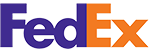•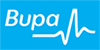•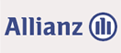•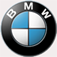•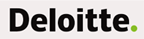•••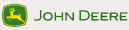•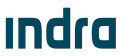•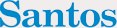•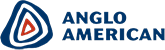•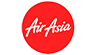•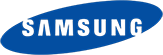•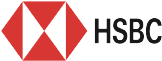••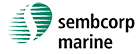•••### Awards and Accreditations

••••